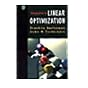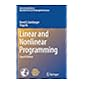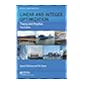Normal view

# Linear and integer optimization: theory and practice

Material type:TextPublisher: Boca Raton CRC Press 2015Edition: 3rd edDescription: xxix, 684 p.ISBN: 9781498710169DDC classification: 519.72 Summary: Presenting a strong and clear relationship between theory and practice, Linear and Integer Optimization: Theory and Practice is divided into two main parts. The first covers the theory of linear and integer optimization, including both basic and advanced topics. Dantzig’s simplex algorithm, duality, sensitivity analysis, integer optimization models, and network models are introduced. More advanced topics also are presented including interior point algorithms, the branch-and-bound algorithm, cutting planes, complexity, standard combinatorial optimization models, the assignment problem, minimum cost flow, and the maximum flow/minimum cut theorem. The second part applies theory through real-world case studies. The authors discuss advanced techniques such as column generation, multiobjective optimization, dynamic optimization, machine learning (support vector machines), combinatorial optimization, approximation algorithms, and game theory. Besides the fresh new layout and completely redesigned figures, this new edition incorporates modern examples and applications of linear optimization. The book now includes computer code in the form of models in the GNU Mathematical Programming Language (GMPL). The models and corresponding data files are available for download and can be readily solved using the provided online solver. This new edition also contains appendices covering mathematical proofs, linear algebra, graph theory, convexity, and nonlinear optimization. All chapters contain extensive examples and exercises. This textbook is ideal for courses for advanced undergraduate and graduate students in various fields including mathematics, computer science, industrial engineering, operations research, and management science. https://www.crcpress.com/Linear-and-Integer-Optimization-Theory-and-Practice-Third-Edition/Sierksma-Zwols/p/book/9781498710169
Tags from this library: No tags from this library for this title.Average rating: 0.0 (0 votes)
Item type Current location Item location Collection Call number Status Date due Barcode
Books Vikram Sarabhai Library
General Stacks
Slot 1682 (2 Floor, East Wing) Non-fiction 519.72 S4L4-2015 (Browse shelf) Available 192908
##### Browsing Vikram Sarabhai Library shelves, Shelving location: General Stacks, Collection: Non-fiction Close shelf browserNo cover image available519.72 B3I6 Introduction to linear optimization 519.72 L8L4 Linear and nonlinear programming 519.72 S2T4 Theory of linear and integer programming 519.72 S4L4-2015 Linear and integer optimization: theory and practice 519.72 S9L4 Linear programming: basic theory and application 519.76 C3Q8 The quadratic assignment problem: theory and algorithms 519.76 C6C6 Combinatorial optimization

Basic Concepts of Linear Optimization
The Company Dovetail
Definition of an LO-Model
Alternatives of the Standard LO-Model
Solving LO-Models Using a Computer Package
Linearizing Nonlinear Functions
Examples of Linear Optimization Models
Building and Implementing Mathematical Models
Exercises

LINEAR OPTIMIZATION THEORY: BASIC TECHNIQUES

Geometry and Algebra of Feasible Regions
The Geometry of Feasible Regions
Algebra of Feasible Regions; Feasible Basic Solutions
Exercises

Dantzig’s Simplex Algorithm
From Vertex to Vertex to an Optimal Solution
LO-Model Reformulation
The Simplex Algorithm
Simplex Tableaus
Discussion of the Simplex Algorithm
Initialization
Uniqueness and Multiple Optimal Solutions
Models with Equality Constraints
The Revised Simplex Algorithm
Exercises

Duality, Feasibility, and Optimality
The Companies Dovetail and Salmonnose
Duality and Optimality
Complementary Slackness Relations
Infeasibility and Unboundedness; Farkas’ Lemma
Primal and Dual Feasible Basic Solutions
Duality and the Simplex Algorithm
The Dual Simplex Algorithm
Exercises

Sensitivity Analysis
Sensitivity of Model Parameters
Perturbing Objective Coefficients
Perturbing Right Hand Side Values (Nondegenerate Case)
Piecewise Linearity of Perturbation Functions
Perturbation of the Technology Matrix
Sensitivity Analysis for the Degenerate Case
Shadow Prices and Redundancy of Equality Constraints
Exercises

Large-Scale Linear Optimization
The Interior Path
Formulation of the Interior Path Algorithm
Convergence to the Interior Path; Maintaining Feasibility
Termination and Initialization
Exercises

Integer Linear Optimization
Introduction
The Branch-and-Bound Algorithm
Linearizing Logical Forms with Binary Variables
Gomory’s Cutting-Plane Algorithm
Exercises

Linear Network Models
LO-Models with Integer Solutions; Total Unimodularity
ILO-Models with Totally Unimodular Matrices
The Network Simplex Algorithm
Exercises

Computational Complexity
Introduction to Computational Complexity
Computational Aspects of Dantzig’s Simplex Algorithm
The Interior Path Algorithm Has Polynomial Running Time
Computational Aspects of the Branch-and-Bound Algorithm
Exercises

Designing a Reservoir for Irrigation
The Parameters and the Input Data
Maximizing the Irrigation Area
Changing the Input Parameters of the Model
GMPL Model Code
Exercises

Classifying Documents by Language
Machine Learning
Classifying Documents Using Separating Hyperplanes
LO-Model for Finding Separating Hyperplane
Validation of a Classifier
Robustness of Separating Hyperplanes; Separation Width
Models that Maximize the Separation Width
GMPL Model Code
Exercises

Production Planning; A Single Product Case
Model Description
Regular Working Hours
Overtime
Allowing Overtime and Idle Time
Sensitivity Analysis
GMPL Model Code
Exercises

Production of Coffee Machines
Problem Setting
An LO-Model that Minimizes Backlogs
Old and Recent Backlogs
Full Week Productions
Sensitivity Analysis
GMPL Model Code
Exercises

Conflicting Objectives: Producing Versus Importing
Problem Description and Input Data
Modeling Two Conflicting Objectives; Pareto Optimal Point
Goal Optimization for Conflicting Objective
Soft and Hard Constraints
Sensitivity Analysis
Alternative Solution Techniques
A Comparison of the Solutions
GMPL Model Code
Exercises

Coalition Formation and Profit Distribution
The Farmers Cooperation Problem
Game Theory; Linear Production Games
How to Distribute the Total Profit Among the Farmers?
Profit Distribution for Arbitrary Numbers of Farmers
Sensitivity Analysis
Exercises

Minimizing Trimloss When Cutting Cardboard
Formulating the Problem
Gilmore-Gomory’s Solution Algorithm
Calculating an Optimal Solution
Exercises

Off-Shore Helicopter Routing
Problem Description
Vehicle Routing Problems
Problem Formulation
ILO Formulation
Column Generation
Dual Values as Price Indicators for Crew Exchanges
A Round-Off Procedure for Determining an Integer Solution
Computational Experiments
Sensitivity Analysis
Exercises

The Catering Service Problem
Formulation of the Problem
The Transshipment Problem Formulation
Applying the Network Simplex Algorithm
Sensitivity Analysis
GMPL Model Code
Exercises

Appendix A Mathematical Proofs
Appendix B Linear Algebra
Appendix C Graph Theory
Appendix D Convexity
Appendix E Nonlinear Optimization
Appendix F Writing LO-Models in GNU MathProg (GMPL)

Presenting a strong and clear relationship between theory and practice, Linear and Integer Optimization: Theory and Practice is divided into two main parts. The first covers the theory of linear and integer optimization, including both basic and advanced topics. Dantzig’s simplex algorithm, duality, sensitivity analysis, integer optimization models, and network models are introduced.
More advanced topics also are presented including interior point algorithms, the branch-and-bound algorithm, cutting planes, complexity, standard combinatorial optimization models, the assignment problem, minimum cost flow, and the maximum flow/minimum cut theorem.
The second part applies theory through real-world case studies. The authors discuss advanced techniques such as column generation, multiobjective optimization, dynamic optimization, machine learning (support vector machines), combinatorial optimization, approximation algorithms, and game theory.
Besides the fresh new layout and completely redesigned figures, this new edition incorporates modern examples and applications of linear optimization. The book now includes computer code in the form of models in the GNU Mathematical Programming Language (GMPL). The models and corresponding data files are available for download and can be readily solved using the provided online solver.
This new edition also contains appendices covering mathematical proofs, linear algebra, graph theory, convexity, and nonlinear optimization. All chapters contain extensive examples and exercises. This textbook is ideal for courses for advanced undergraduate and graduate students in various fields including mathematics, computer science, industrial engineering, operations research, and management science.

https://www.crcpress.com/Linear-and-Integer-Optimization-Theory-and-Practice-Third-Edition/Sierksma-Zwols/p/book/9781498710169

There are no comments for this item.